# Laplacian Edge Detection

We wish to build a morphing algorithm which operates on features automatically extracted from target images. A good beginning is to find the edges in the target images. We accomplished this by implementing a Laplacian Edge Detector.Step 1: Start with an image of a good looking team member. Since no such images were available, we used the image shown to the right. Step 2: Blur the image. Since we want to select edges to perform a morph, we don't really need "every" edge in the image, only the main features. Thus, we blur the image prior to edge detection. This blurring is accomplished by convolving the image with a gaussian (A gaussian is used because it is "smooth"; a general low pass filter has ripples, and ripples show up as edges) Step 3: Perform the laplacian on this blurred image. Why do we use the laplacian? Let's look at an example in one dimension. Suppose we have the following signal, with an edge as highlited below.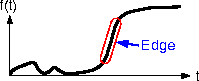If we take the gradient of this signal (which, in one dimension, is just the first derivative with respect to t) we get the following: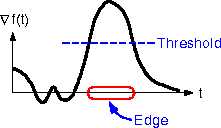Clearly, the gradient has a large peak centered around the edge. By comparing the gradient to a threshold, we can detect an edge whenever the threshold is exceeded (as shown above). In this case, we have found the edge, but the edge has become "thick" due to the thresholding. However, since we know the edge occurs at the peak, we can localize it by computing the laplacian (in one dimension, the second derivative with respect to t) and finding the zero crossings.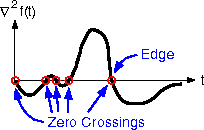The above figure shows the laplacian of our one-dimensional signal. As expected, our edge corresponds to a zero crossing, but we also see other zero crossings which correspond to small ripples in the original signal. When we apply the laplacian to our test image, we get the following: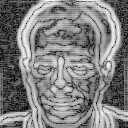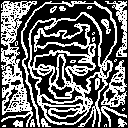The left image is the log of the magnitude of the laplacian, so the dark areas correspond to zeros. The right image is a binary image of the zero crossings of the laplacian. As expected, we have found the edges of the test image, but we also have many false edges due to ripple and texture in the image.To remove these false edges, we add a step to our algorithm. When we find a zero crossing of the laplacian, we must also compute an estimate of the local variance of the test image, since a true edge corresponds to a significant change in intensity of the original image. If this variance is low, then our zero crossing must have been caused by ripple. Thus, we have Step 4: Find the zero crossings of the laplacian and compare the local variance at this point to a threshold. If the threshold is exceeded, declare an edge. The result of this step is shown to the right. And finally, we have Step 5: Median Filter the image. We apply a median filter because it removes the spot noise while preserving the edges. This yields a very clean representation of the major edges of the original image, as shown below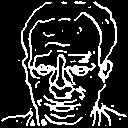We are now ready to extract some morphing features!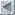Click here to go to the automatic morphing pageClick here to go to other methods of edge detectionClick here to return to the main morph page